Documentation

# comm.EyeDiagram

Display eye diagram of time-domain signals

## Description

The eye diagram System object™ displays multiple traces of a modulated signal to produce an eye diagram. You can use the object to reveal the modulation characteristics of the signal, such as the effects of pulse shaping or channel distortions. The eye diagram can measure signal characteristics and plot horizontal and vertical bathtub curves when the jitter and noise comply with the dual-Dirac model .

To display the eye diagram of an input signal:

1. Create a `comm.EyeDiagram` object and set the properties of the object.

2. Call `step` to display the eye diagram of the signal.

### Note

Alternatively, instead of using the `step` method to perform the operation defined by the System object, you can call the object with arguments, as if it were a function. For example, `y = step(obj,x)` and `y = obj(x)` perform equivalent operations.

## Construction

`ed = comm.EyeDiagram` returns an eye diagram object, `ed`, using the default properties.

`ed = comm.EyeDiagram(Name,Value)` specifies additional properties using `Name,Value` pairs. Unspecified properties have default values.

Example:

```ed = comm.EyeDiagram('DisplayMode','2D color histogram', ... 'OversamplingMethod','Input interpolation');```

## Properties

expand all

Name displayed on the eye diagram window, specified as a character vector. Tunable.

Sample rate of the input signal in Hz, specified as a positive real scalar.

Number of samples per symbol, specified as a positive integer scalar. Tunable.

Number of samples to omit before plotting the first point, specified as a nonnegative integer scalar. To avoid irregular behavior, specify the offset to be less than the product of `SamplesPerSymbol` and `SamplePerTrace`.

Number of symbols per trace, specified as a positive integer scalar. To obtain eye measurements and visualize bathtub curves, use the default value of `2`. Tunable.

Number of traces to display, specified as a positive integer scalar. This property is available when the `DisplayMode` property is specified as `'Line plot'`. Tunable.

Eye diagram display mode, specified as `'Line plot'` or `'2D color histogram'`. Tunable.

• Specify `'Line plot'` to overlay traces by plotting one line for each of the last `TracesToDisplay` traces.

• Specify `'2D color histogram'` to display a color gradient that shows how often the input matches different time and amplitude values.

Enable eye diagram measurements, specified as a logical scalar. Tunable.

Enable visualization of bathtub curves, specified as `'None'`, `'Horizontal'`, `'Vertical'`, or `'Both'`. This property is available when `EnableMeasurements` is `true`. Tunable.

Histogram overlay, specified as `'None'`, `'Jitter'`, or `'Noise'`.

• To overlay a horizontal histogram on the eye diagram, set this property to `'Jitter'`.

• To overlay a vertical histogram on the eye diagram, set this property to `'Noise'`.

This property is available when `DisplayMode` is ```'2D color histogram'``` and `EnableMeasurements` is `true`. Tunable.

Amplitude level threshold in V, specified as a scalar. This property separates the different signaling regions for horizontal (jitter) histograms, and is available when `EnableMeasurements` is `true`. This property is tunable, but the jitter histograms reset when the property changes.

For non-return-to-zero (NRZ) signals, set `DecisionBoundary` to 0. For return-to-zero (RZ) signals, set `DecisionBoundary` to half the maximum amplitude.

Time range for calculating eye levels, specified as a two-element vector. These values are expressed as a percentage of the symbol duration. This property is available when `EnableMeasurements` is `true`. Tunable.

Amplitude levels of the rise and fall transitions, specified as a two-element vector. These values are expressed as a percentage of the eye amplitude. This property is available when `EnableMeasurements` is `true`. This property is tunable but the crossing histograms of the rise and fall thresholds reset when it is changed.

Amplitude tolerance of the horizontal crossings in V, specified as a scalar. Increase hysteresis to provide more tolerance to spurious crossings due to noise. This property is available when `EnableMeasurements` is `true`. This property is tunable, but the jitter and the rise and fall histograms reset when the property changes.

BER used for eye measurements, specified as a nonnegative scalar from 0 to 0.5. The value is used to make measurements of random jitter, total jitter, horizontal eye openings, and vertical eye openings. This property is available when `EnableMeasurements` is `true`. Tunable.

BER values used to calculate openings of bathtub curves, specified as a vector whose elements range from 0 to 0.5. Horizontal and vertical eye openings are calculated for each of the values specified by this property. This property is available when `EnableMeasurements` is `true` and `ShowBathtub` is `'Both'`, `'Horizontal'`, or `'Vertical'`. Tunable.

Duration of initial data discarded from measurements in seconds, specified as a nonnegative scalar. This property is available when `EnableMeasurements` is `true`.

Oversampling method, specified as `'None'`, ```'Input interpolation'```, or `'Histogram interpolation'`. This property is available when `DisplayMode` is ```'2D color histogram'```. Tunable.

To plot eye diagrams as quickly as possible, set `OversamplingMethod` to `'None'`. The drawback to not oversampling is that the plots look pixelated when the number of samples per trace is small.

To create smoother, less-pixelated plots using a small number of samples per trace, set `OversamplingMethod` to`'Input interpolation'` or `'Histogram interpolation'`. Input interpolation is the faster interpolation method and produces good results when the signal-to-noise ratio (SNR) is high. With a lower SNR, this oversampling method is not recommended because it introduces a bias to the centers of the histogram ranges. Histogram interpolation is not as fast as the other techniques, but it provides good results even when the SNR is low.

Color scale of the histogram, specified as `'Linear'` or `'Logarithmic'`. Change this property if certain areas of the histogram include a disproportionate number of points. Use the `'Logarithmic'` option for eye diagrams having sharp peaks, where the signal repetitively matches specific time and amplitude values. This property is available when `DisplayMode` is ```'2D color histogram'```. Tunable.

Color fading, specified as a logical scalar. To fade the points in the display as the interval of time after they are first plotted increases, set this property to `true`. This animation resembles an oscilloscope. This property is available when  `DisplayMode` is ```'Line plot'```. Tunable.

Show imaginary signal component, specified as a logical scalar. To view the imaginary or quadrature component of the input signal, set this property to `true`. This property is available when `EnableMeasurements` is `false`. Tunable.

Y-axis limits of the eye diagram in V, specified as a two-element vector. The first element corresponds to ymin and the second to ymax. The second element must be greater than the first. Tunable.

Enable grid display on eye diagram, specified as a logical scalar. To display a grid on the eye diagram, set this property to `true`. Tunable.

Scope window position in pixels, specified as a four-element vector of the form `[left bottom width height]`. Tunable.

## Methods

 hide Hide scope window horizontalBathtub Horizontal bathtub curve jitterHistogram Jitter histogram measurements Measure eye diagram parameters noiseHistogram Noise histogram reset Reset states of eye diagram object show Make scope window visible step Plot eye diagram of input signal verticalBathtub Vertical bathtub curve
Common to All System Objects
`release`

Allow System object property value changes

## Examples

expand all

Specify the sample rate and the number of output samples per symbol parameters.

```fs = 1000; sps = 4; ```

Create transmit filter and eye diagram objects.

```txfilter = comm.RaisedCosineTransmitFilter('OutputSamplesPerSymbol',sps); ed = comm.EyeDiagram('SampleRate',fs*sps,'SamplesPerSymbol',sps); ```

Generate random symbols and apply QPSK modulation. Then filter the modulated signal and display the eye diagram.

```data = randi([0 3],1000,1); modSig = pskmod(data,4,pi/4); txSig = txfilter(modSig); ed(txSig) ```Show the effects of different interpolation methods on 2-D histograms for different signal-to-noise ratio (SNR) conditions.

Create GMSK modulator and eye diagram System objects. Specify that the eye diagram displays using a 2-D color histogram and plots the real and imaginary signals.

```gmsk = comm.GMSKModulator('PulseLength',3); ed = comm.EyeDiagram('DisplayMode','2D color histogram', ... 'ShowImaginaryEye',true,'YLimits',[-2 2]); ```

Generate bipolar data and apply GMSK modulation.

```d = 2*randi([0 1],1e4,1)-1; x = gmsk(d); ```
```%Pass the signal through an AWGN channel having a 25 dB SNR and with a fixed seed for repeatable results. randStream = RandStream('mt19937ar','Seed',5489); y = awgn(x,25,'measured',randStream); ```

Display the eye diagram.

```ed(y) ```For a small number of samples per trace (16), the lack of interpolation causes piecewise-continuous behavior.

To compensate for the piecewise-continuous behavior, set the `OversamplingMethod` property to `'Input interpolation'`. Reset the object and display the eye diagram.

```ed.OversamplingMethod = 'Input interpolation'; reset(ed) ed(y) ```The interpolation smooths the eye diagram.

Now pass the GMSK-modulated signal through an AWGN channel having a 10 dB SNR. Display the eye diagram.

```y = awgn(x,10,'measured',randStream); reset(ed) ed(y) ```The vertical striping is the result of input interpolation, which has limited accuracy in low-SNR conditions.

Set the `OversamplingMethod` property to `'Histogram interpolation'`. Plot the eye diagram.

```ed.OversamplingMethod = 'Histogram interpolation'; reset(ed) ed(y) ```The eye diagram plot now renders accurately because the histogram interpolation method works for all SNR values. This method results in increased execution time.

Visualize the eye diagram of a dual-dirac input signal. Compute eye measurements, and visualize horizontal and vertical bathtub curves. Overlay the horizontal (jitter) histogram.

Specify the sample rate, the samples per symbol, and the number of traces.

```fs = 10000; sps = 200; numTraces = 2000; ```

Create an eye diagram object having these properties:

• 2-D color histogram display

• Logarithmic color scale

• Jitter histogram overlay

• Horizontal and vertical bathtub curves

• Y-axis limits of [-1.3 1.3]

• Increased window height

```ed = comm.EyeDiagram('SampleRate',fs,'SamplesPerSymbol',sps,'SampleOffset',sps/2, ... 'DisplayMode','2D color histogram','ColorScale','Logarithmic', ... 'EnableMeasurements',true,'OverlayHistogram','Jitter', ... 'ShowBathtub','Both','YLimits', [-1.3 1.3]); ed.Position = ed.Position + [0 0 0 120]; ```

Generate a waveform having dual-dirac and random jitter. Specify 3 ms rise and fall times.

```src = commsrc.pattern('SamplesPerSymbol',sps,'RiseTime',3e-3,'FallTime', 3e-3); src.Jitter = commsrc.combinedjitter('RandomJitter','on','DiracJitter','on', ... 'DiracDelta',[-10e-04 10e-04],'RandomStd',5e-4); ```

Generate two symbols for each trace.

```symbols = src.generate(numTraces*2); ```

Process the data in packets of 40e3 symbols, add noise, and display the eye diagram.

```for idx = 1:(numTraces-1)/100 x = symbols(1+(idx-1)*100*2*sps:idx*100*2*sps); % Read 40,000 symbols y = awgn(x,30); % Add noise ed(y); % Display eye diagram end ```Display the eye diagram for a waveform having dual-dirac and random jitter. Plot the jitter and noise histograms.

Specify the sample rate, the samples per symbol, and the number of traces parameters.

```fs = 1000; sps = 200; numTraces = 1000; ```

Create an eye diagram object.

```ed = comm.EyeDiagram('SampleRate',fs,'SamplesPerSymbol',sps,'SampleOffset',sps/2, ... 'DisplayMode','2D color histogram','ColorScale','Logarithmic', ... 'EnableMeasurements',true,'YLimits',[-1.2 1.2]); ```

Generate a waveform having dual-dirac and random jitter. Specify 3 ms rise and fall times.

```src = commsrc.pattern('SamplesPerSymbol',sps,'RiseTime',3e-3,'FallTime', 3e-3); src.Jitter = commsrc.combinedjitter('RandomJitter','on','DiracJitter','on', ... 'DiracDelta',[-10e-04 10e-04],'RandomStd',5e-4); ```

Generate two symbols for each trace.

```x = src.generate(numTraces*2); ```

Pass the signal through an AWGN channel with a fixed seed for repeatable results.

```randStream = RandStream('mt19937ar','Seed',5489); y = awgn(x,30,'measured',randStream); ed(y) ```Calculate the jitter histogram count for each bin by using the `jitterHistogram` method. Plot the histogram.

```jbins = jitterHistogram(ed); plot(jbins) ```Calculate the noise histogram count for each bin by using the `noiseHistogram` method. Plot the histogram.

```nbins = noiseHistogram(ed); plot(nbins) ```Display the eye diagram for a waveform having dual-dirac and random jitter. Generate and plot the horizontal and vertical bathtub curves.

Specify the sample rate, the samples per symbol, and the number of traces parameters.

```fs = 1000; sps = 200; numTraces = 1000; ```

Create an eye diagram object.

```ed = comm.EyeDiagram('SampleRate',fs,'SamplesPerSymbol',sps,'SampleOffset',sps/2, ... 'DisplayMode','2D color histogram','ColorScale','Logarithmic', ... 'EnableMeasurements',true,'ShowBathtub','Both','YLimits',[-1.2 1.2]); ```

Generate a waveform having dual-dirac and random jitter. Specify 3 ms rise and fall times.

```src = commsrc.pattern('SamplesPerSymbol',sps,'RiseTime',3e-3,'FallTime', 3e-3); src.Jitter = commsrc.combinedjitter('RandomJitter','on','DiracJitter','on', ... 'DiracDelta',[-5e-04 5e-04],'RandomStd',2e-4); ```

Generate two symbols for each trace.

```x = src.generate(numTraces*2); ```

Pass the signal through an AWGN channel with a fixed seed for repeatable results.

```randStream = RandStream('mt19937ar','Seed',5489); y = awgn(x,30,'measured',randStream); ```

Display the eye diagram.

```ed(y) ```Generate the horizontal bathtub data for the eye diagram. Plot the curve.

```hb = horizontalBathtub(ed) semilogy([hb.LeftThreshold],[hb.BER],'b',[hb.RightThreshold],[hb.BER],'b') grid ```
```hb = 1x13 struct array with fields: BER LeftThreshold RightThreshold ```Generate the vertical bathtub data for the eye diagram. Plot the curve.

```vb = verticalBathtub(ed) semilogx([vb.BER],[vb.LowerThreshold],'b',[vb.BER],[vb.UpperThreshold],'b') grid ```
```vb = 1x13 struct array with fields: BER UpperThreshold LowerThreshold ```Create a combined jitter object having random jitter with a 2e-4 standard deviation.

```jtr = commsrc.combinedjitter('RandomJitter','on','RandomStd',2e-4); ```

Generate an NRZ signal having random jitter and 3 ms rise and fall times.

```genNRZ = commsrc.pattern('Jitter',jtr,'RiseTime',3e-3,'FallTime',3e-3); x = generate(genNRZ,2000); ```

Pass the signal through an AWGN channel with fixed seed for repeatable results.

```randStream = RandStream('mt19937ar','Seed',5489); y = awgn(x,30,'measured',randStream); ```

Create an eye diagram object. Enable the measurements.

```ed = comm.EyeDiagram('SamplesPerSymbol',genNRZ.SamplesPerSymbol, ... 'SampleRate',genNRZ.SamplingFrequency,'SampleOffset',genNRZ.SamplesPerSymbol/2, ... 'EnableMeasurements',true,'DisplayMode','2D color histogram', ... 'OversamplingMethod','Input interpolation','ColorScale','Logarithmic','YLimits',[-1.2 1.2]); ```

To compute the rise and fall times, determine the rise and fall thresholds from the eye level and eye amplitude measurements. Plot the eye diagram to calculate these parameters.

```ed(y) ```Pass the signal through the eye diagram object again to measure the rise and fall times.

```ed(y) hide(ed) ```

Display the data by using the `measurements` method.

```eyestats = measurements(ed); riseTime = eyestats.RiseTime fallTime = eyestats.FallTime ```
```riseTime = 0.0030 fallTime = 0.0030 ```

The measured values match the 3 ms specification.

## Measurements

expand all

To open the measurements panel, click on the button or select Tools > Measurements > Eye Measurements from the toolbar menu.

### Note

• For amplitude measurements, at least one bin per vertical histogram must reach 10 hits before the measurement is taken, ensuring higher accuracy.

• For time measurements, at least one bin per horizontal histogram must reach 10 hits before the measurement is taken.

• When an eye crossing time measurement falls within the [-0.5/Fs, 0) seconds interval, the time measurement wraps to the end of the eye diagram, i.e., the measurement wraps by 2*Ts seconds (where Ts is the symbol time). For a complex signal case, the analyze method issues a warning if the crossing time measurement of the in-phase branch wraps while that of the quadrature branch does not (or vice versa). To avoid the time-wrapping or a warning, add a half-symbol duration delay to the current value in the `MeasurementDelay` property of the eye diagram object. This additional delay repositions the eye in the approximate center of the scope.

Eye level is the amplitude level used to represent data bits. For the displayed NRZ signal, the levels are –1 V and +1 V. The eye levels are calculated by averaging the 2-D histogram within the eye level boundaries.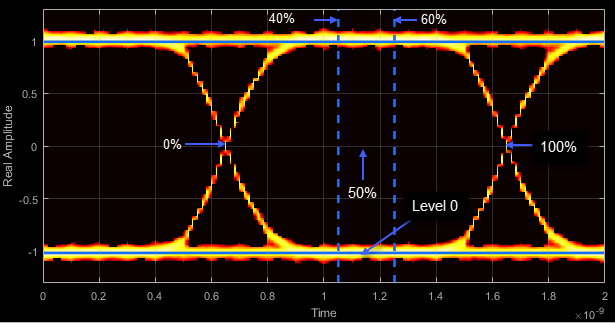Eye amplitude is the distance in V between the mean value of two eye levels.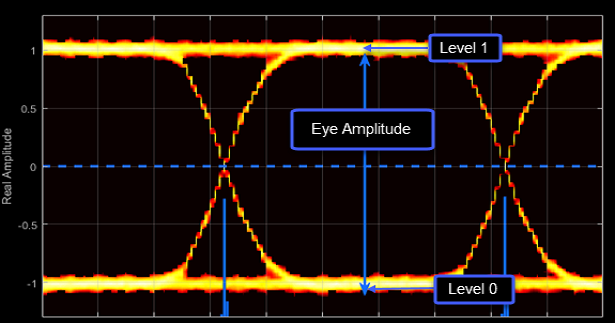Eye height is the distance between μ – 3σ of the upper eye level and μ + 3σ of the lower eye level. μ is the mean of the eye level and σ is the standard deviation.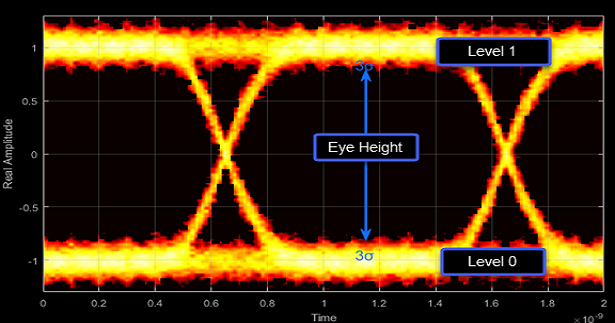The vertical opening is the distance between the two points that correspond to the BER threshold. For example, for a BER threshold of 10–12, these points correspond to the 7σ distance from each eye level.The eye SNR is the ratio of the eye level difference to the difference of the vertical standard deviations corresponding to each eye level:

`$\text{SNR}=\frac{{L}_{1}-{L}_{0}}{{\sigma }_{1}-{\sigma }_{0}}\text{\hspace{0.17em}},$`

where L1 and L0 represent the means of the upper and lower eye levels and σ1 and σ0 represent their standard deviations.

The Q factor is calculated using the same formula as the Eye SNR. However, the standard deviations of the vertical histograms are replaced with those computed with the dual-Dirac analysis.

The crossing levels are the amplitude levels at which the eye crossings occur.The crossing times are the times at which the crossings occur. The times are computed as the mean values of the horizontal (jitter) histograms.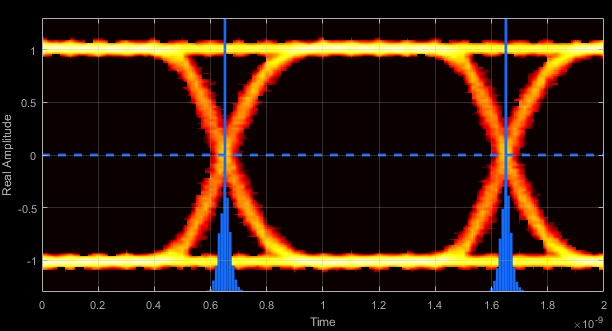Eye delay is the midpoint between the two crossing times.Eye width is the horizontal distance between μ + 3σ of the left crossing time and μ – 3σ of the right crossing time. μ is the mean of the jitter histogram and σ is the standard deviation.The horizontal opening is the distance between the two points that correspond to the BER threshold. For example, for a 10–12 BER, these two points correspond to the 7σ distance from each crossing time.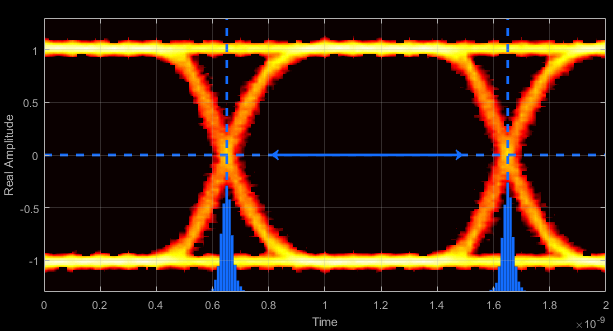Rise time is the mean time between the low and high thresholds defined in the eye diagram. The default thresholds are 10% and 90% of the eye amplitude.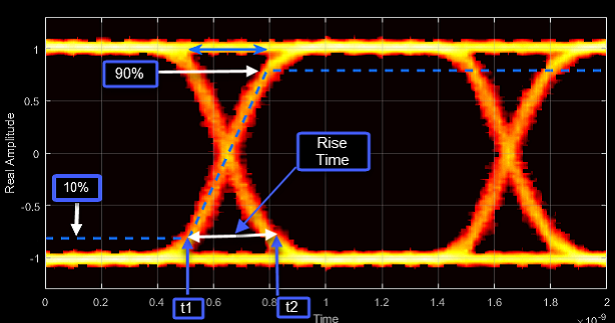Fall time is the mean time between the high and low thresholds defined in the eye diagram. The default thresholds are 10% and 90% of the eye amplitude.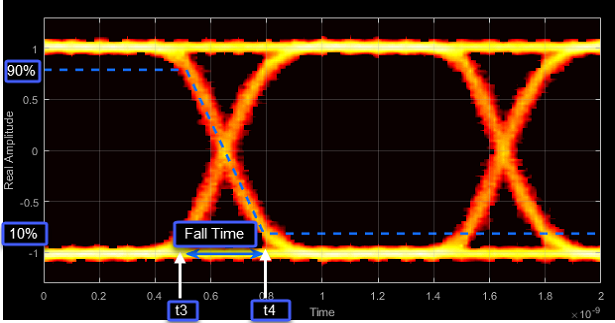The deterministic jitter (DJ) is the distance between the two peaks of the dual-Dirac histograms. The probability density function (PDF) of DJ is composed of two delta functions.The random jitter (RJ) is the Gaussian unbounded jitter component. The random component of jitter is modeled as a zero-mean Gaussian random variable with a specified standard-deviation, σ. The random jitter is computed as:

`$\text{RJ}=\left({Q}_{L}+{Q}_{R}\right)\sigma \text{\hspace{0.17em}},$`

where

`$Q=\sqrt{2}{\mathrm{erfc}}^{-1}\left(2\frac{BER}{\rho }\right)\text{\hspace{0.17em}}.$`

BER is the specified BER threshold. ρ is the amplitude of the left and right Dirac function, which is determined from the bin counts of the jitter histograms.Total jitter (TJ) is the sum of the deterministic and random jitter, such that TJ = DJ + RJ.The total jitter PDF is the convolution of the DJ PDF and the RJ PDF.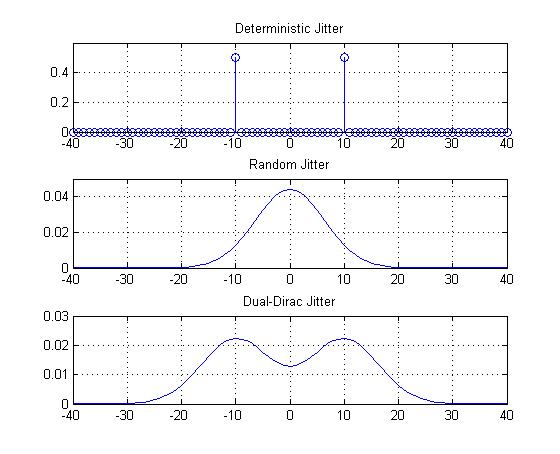RMS jitter is the standard deviation of the jitter calculated in the horizontal (jitter) histogram at the decision boundary.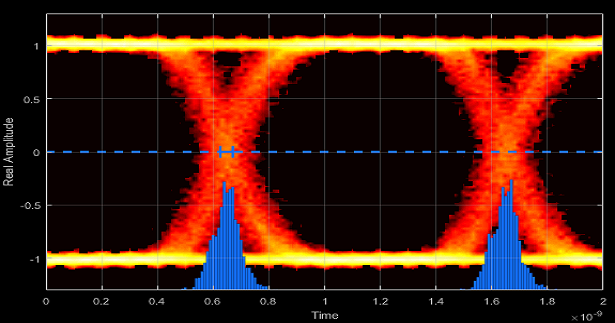Peak-to-peak jitter is the maximum horizontal distance between the left and right nonzero values in the horizontal histogram of each crossing time.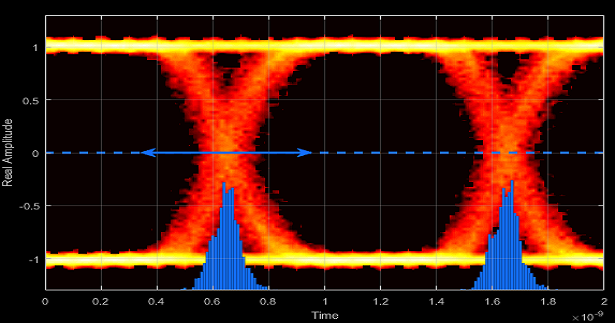## Programmatic Configuration

You can programmatically configure the scope properties with callbacks or within scripts by using a scope configuration object as describe in Control Scope Blocks Programmatically (Simulink).

 Stephens, Ransom. "Jitter Analysis: The Dual-Dirac Model, RJ/DJ, and Q-Scale." Agilent Technologies Whitepaper. December 2004.